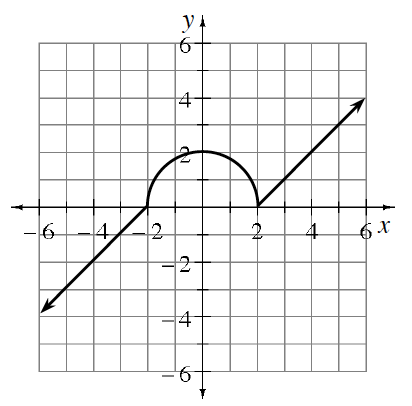### Home > INT3 > Chapter 4 > Lesson 4.4.3 > Problem4-131

4-131.

Given the graph of $y=f(x)$ at right, sketch each of the following transformations.

1. $y=-2f(x)$

Pick out key points on the graph. Multiply the $y$-coordinate of each point by $−2$ and graph the new point.

2. $y=f(x-1)-3$

Translate the graph right $1$ unit and down $3$ units.

3. $y=f(-x)$

Think about a blank graph. How are the positive $x$-values related to the negative $x$-values?